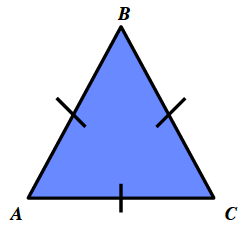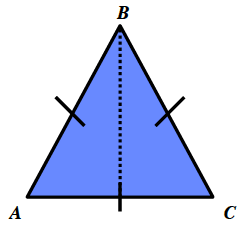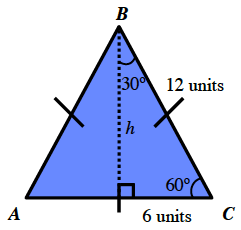### Home > GC > Chapter 7 > Lesson 7.2.4 > Problem7-72

7-72.

Use Tromika's method from problem 7-68 to find the area of an equilateral triangle with side length 12 units. Show all work. Homework Help ✎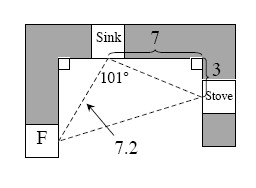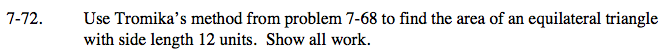Draw the triangle described.

Draw a line which bisects the triangle.

Find the measure of the two acute angles and the length of the shorter leg in one of the two right triangles formed by the division of the equilateral triangle.

Use trigonometry to find the height.

$\text{cos}\angle B \frac{h}{12}\ \ \ \text{sin}\angle C\frac{h}{12}\ \ \ \text{tan}\angle C\frac{h}{6}\ \ \ \text{tan}\angle B\frac{6}{h}$

h ≈ 10.39

Use the height to find the area of the triangle.

$\left ( \frac{1}{2} \right )(12\text{ units})(10.39\text{ units})\approx 62.34 \text{ units}^{2}$